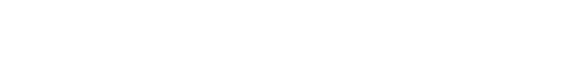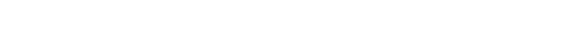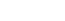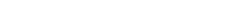BERT蒸馏有什幺技巧？如何调参？### 模型蒸馏原理

Hinton在NIPS2014  提出了知识蒸馏（Knowledge Distillation）的概念，旨在把一个大模型或者多个模型ensemble学到的知识迁移到另一个轻量级单模型上，方便部署。简单的说就是用小模型去学习大模型的预测结果，而不是直接学习训练集中的label。

### 如何蒸馏### BERT蒸馏

Distilled BiLSTM

Distilled BiLSTM  于2019年5月提出，作者将BERT-large蒸馏到了单层的BiLSTM中，参数量减少了100倍，速度提升了15倍，效果虽然比BERT差不少，但可以和ELMo打成平手。Distilled BiLSTM的教师模型采用精调过的BERT-large，学生模型采用BiLSTM+ReLU，蒸馏的目标是hard labe的交叉熵+logits之间的MSE（作者经过实验发现MSE比上文的更好）。

### BERT-PKD (EMNLP2019)

BERT-PKD  不同于之前的研究，提出了 Patient Knowledge Distillation ，即从教师模型的中间层提取知识，避免在蒸馏最后一层时拟合过快的现象（有过拟合的风险）。### DIstillBERT (NIPS2019）

DistillBERT的教师模型采用了预训练好的BERT-base，学生模型则是6层transformer，采用了PKD-skip的方式进行初始化。和之前蒸馏目标不同的是，为了调整教师和学生的隐层向量方向，作者新增了一个cosine embedding loss，蒸馏最后一层hidden的。最终 损失函数由MLM loss、-学生最后一层的交叉熵、隐层之间的cosine loss组成 。从消融实验可以看出，MLM loss对于学生模型的表现影响较小，同时初始化也是影响效果的重要因素：### TinyBERT（EMNLP2019）

TinyBERT  就提出了two-stage learning框架，分别在预训练和精调阶段蒸馏教师模型，得到了参数量减少7.5倍，速度提升9.4倍的4层BERT，效果可以达到教师模型的96.8%，同时这种方法训出的6层模型甚至接近BERT-base，超过了BERT-PKD和DistillBERT。TinyBERT的教师模型采用BERT-base。作者参考其他研究的结论，即注意力矩阵可以捕获到丰富的知识，提出了注意力矩阵的蒸馏，采用教师-学生注意力矩阵logits的MSE作为损失函数（这里不取attention prob是实验表明前者收敛更快）。另外，作者还对embedding进行了蒸馏，同样是采用MSE作为损失。### MobileBERT（ACL2020）

MobileBERT压缩维度的主要思想在于bottleneck机制，如下图所示：MobileBERT的蒸馏中，作者先用b的结构预训练一个BERT-large，再蒸馏到24层学生模型中。蒸馏的loss有多个：

Feature Map Transfer：隐层的MSE
Attention Transfer：注意力矩阵的KL散度
Pre-training Distillation：MobileBERT还有一点不同于之前的TinyBERT，就是预训练阶段蒸馏之后，作者直接在MobileBERT上用任务数据精调，而不需要再进行精调阶段的蒸馏，方便了很多。

### MiniLMValue-Relation Transfer可以让学生模型更深入地模仿教师模型，实验表明可以带来1-2个点的提升。同时作者考虑到学生模型的层数、维度都可能和教师模型不同，在实验中只蒸馏最后一层，并且 只蒸馏这两个矩阵的KL散度 ，简直是懒癌福音。

### 剪层还是减维度？### 用哪个Loss？### 蒸馏代码实战

```def kd_ce_loss(logits_S, logits_T, temperature=1):
'''
Calculate the cross entropy between logits_S and logits_T
:param logits_S: Tensor of shape (batch_size, length, num_labels) or (batch_size, num_labels)
:param logits_T: Tensor of shape (batch_size, length, num_labels) or (batch_size, num_labels)
:param temperature: A float or a tensor of shape (batch_size, length) or (batch_size,)
'''
if isinstance(temperature, torch.Tensor) and temperature.dim() > 0:
temperature = temperature.unsqueeze(-1)
beta_logits_T = logits_T / temperature
beta_logits_S = logits_S / temperature
p_T = F.softmax(beta_logits_T, dim=-1)
loss = -(p_T * F.log_softmax(beta_logits_S, dim=-1)).sum(dim=-1).mean()
return loss```

```def hid_mse_loss(state_S, state_T, mask=None):
'''
* Calculates the mse loss between `state_S` and `state_T`, which are the hidden state of the models.
* If the hidden sizes of student and teacher are different, 'proj' option is required in `inetermediate_matches` to match the dimensions.
:param torch.Tensor state_S: tensor of shape  (*batch_size*, *length*, *hidden_size*)
:param torch.Tensor state_T: tensor of shape  (*batch_size*, *length*, *hidden_size*)
:param torch.Tensor mask:    tensor of shape  (*batch_size*, *length*)
'''
loss = F.mse_loss(state_S, state_T)
else:
loss = (F.mse_loss(state_S, state_T, reduction='none') * mask.unsqueeze(-1)).sum() / valid_count
return loss```

```def att_mse_loss(attention_S, attention_T, mask=None):
'''
* Calculates the mse loss between `attention_S` and `attention_T`.
:param torch.Tensor logits_S: tensor of shape  (*batch_size*, *num_heads*, *length*, *length*)
:param torch.Tensor logits_T: tensor of shape  (*batch_size*, *num_heads*, *length*, *length*)
:param torch.Tensor mask: tensor of shape  (*batch_size*, *length*)
'''
attention_S_select = torch.where(attention_S <= -1e-3, torch.zeros_like(attention_S), attention_S)
attention_T_select = torch.where(attention_T <= -1e-3, torch.zeros_like(attention_T), attention_T)
loss = F.mse_loss(attention_S_select, attention_T_select)
else:
return loss```

```def cos_loss(state_S, state_T, mask=None):
'''
* Computes the cosine similarity loss between the inputs. This is the loss used in DistilBERT, see `DistilBERT <https://arxiv.org/abs/1910.01108>`_
* If the hidden sizes of student and teacher are different, 'proj' option is required in `inetermediate_matches` to match the dimensions.
:param torch.Tensor state_S: tensor of shape  (*batch_size*, *length*, *hidden_size*)
:param torch.Tensor state_T: tensor of shape  (*batch_size*, *length*, *hidden_size*)
:param torch.Tensor mask:    tensor of shape  (*batch_size*, *length*)
'''
state_S = state_S.view(-1,state_S.size(-1))
state_T = state_T.view(-1,state_T.size(-1))
else:
target = state_S.new(state_S.size(0)).fill_(1)
loss = F.cosine_embedding_loss(state_S, state_T, target, reduction='mean')
return loss```

### 参考资料

 Distilling the Knowledge in a Neural Network: https:// arxiv.org/abs/1503.0253 1

 Distilling Task-Specific Knowledge from BERT into Simple Neural Networks: https:// arxiv.org/abs/1903.1213 6

 Patient Knowledge Distillation for BERT Model Compression: https:// arxiv.org/abs/1908.0935 5

 DistilBERT, a distilled version of BERT: smaller, faster, cheaper and lighter: https:// arxiv.org/abs/1910.0110 8

 TinyBERT: Distilling BERT for Natural Language Understanding: https:// arxiv.org/abs/1909.1035 1

 MobileBERT: a Compact Task-Agnostic BERT for Resource-Limited Devices: https:// arxiv.org/abs/2004.0298 4

 MINILM: Deep Self-Attention Distillation for Task-Agnostic Compression of Pre-Trained Transformers: https:// arxiv.org/abs/2002.1095 7

 TextBrewer: https:// github.com/airaria/Text Brewer

 模型压缩实践收尾篇——模型蒸馏以及其他一些技巧实践小结: https:// zhuanlan.zhihu.com/p/12 4215760